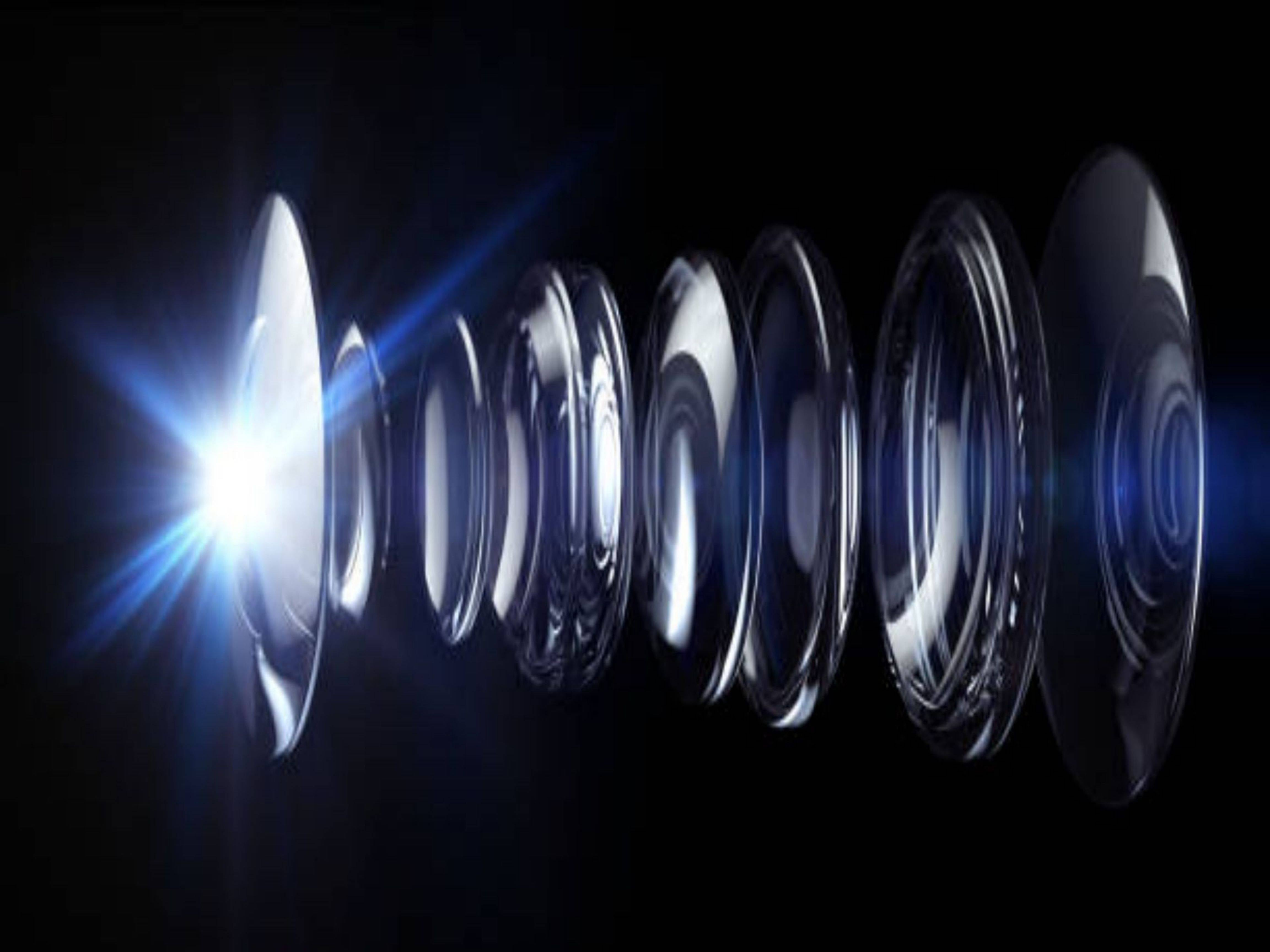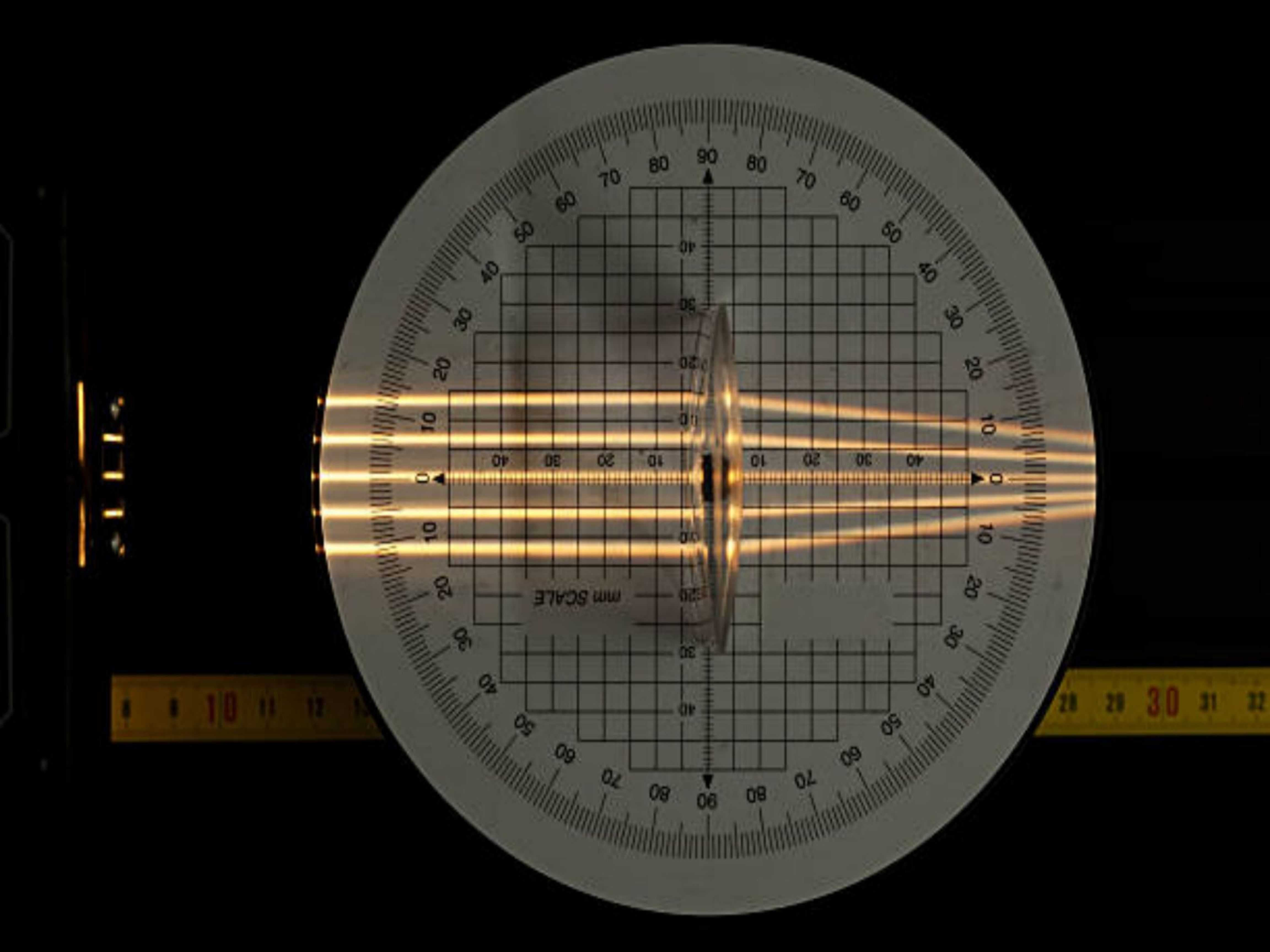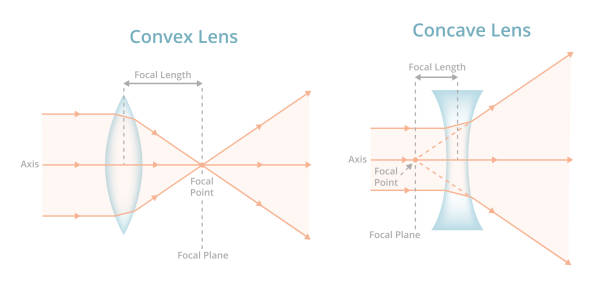# What is the function of a convex lens?

Convex lenses are made according to the principle of refraction of light. A convex lens is a lens that is thicker in the center and thinner at the edges. Convex lenses are divided into bi-convex, plano-convex and concave-convex (or positive meniscus) forms. Convex lenses have a converging effect, so they are also called condensing lenses. Thicker convex lenses have functions such as distance and convergence. The content of the role of convex lens, welcome to read!Convex lenses are clinically called hyperopia or reading glasses, or magnifying glasses, which mainly gather light together. After the object is refracted by the eye, the normal focus should fall on the retina. However, if there is a refractive problem, the focal point after the object is refracted may fall in front of the retina or behind the retina, resulting in nearsightedness or farsightedness. When the focus falls in front of the retina, it is called myopia, and when it falls behind the retina, it is called hyperopia. Hyperopia means that the ability of light to diverge is too strong. At this time, convex lens correction is required. Wearing a convex lens means wearing hyperopia. It concentrates the light and moves the focal point forward, so that the focal point falls exactly on the retina, and distant objects will be sharp. Convex lenses are mainly used to correct farsightedness, or close to correct presbyopia.

Imaging Features:

Convex lenses are refraction imaging, and the imaging can be an inverted, reduced real image; an inverted, equal-sized real image; an inverted, enlarged real image; an upright, enlarged virtual image.
For the role of light: the convex lens is mainly used for the concentration of light.imaging law

1: When the object distance is greater than 2 times the focal length, the object distance is between 1 times the focal length and 2 times the focal length, forming an inverted and reduced real image. At this time, the image distance is smaller than the object distance, the image is smaller than the object, and the object image is on the opposite side. Usage: video camera, video camera.

2: When the object distance is equal to 2 times the focal length, the image distance is also 2 times the focal length, forming an inverted, equal-sized real image. At this time, the object distance is equal to the image distance, the image is equal to the size of the object, and the object is on the opposite side. Application: Measure focal length.

3: When the object distance is less than 2 times the focal length and greater than 1 times the focal length, the image distance is greater than 2 times the focal length, forming an inverted and enlarged real image. At this time, the image distance is greater than the object distance, the image is larger than the object, and the object is on the opposite side. Applications: projectors, slide projectors, movie projectors.

4: When the object distance is equal to 1 times the focal length, it will not be imaged, but parallel light.

5: When the object distance is less than 1 times the focal length, a positive magnified virtual image will be formed. At this time, the image distance is greater than the object distance, the image is larger than the object, and the object image is on the same side. Usage: magnifying glassstructure:

Convex lens: The edge is thin, the middle is thick, at least one side should be spherical, or both sides should be spherical. It can be divided into two types: biconvex, planoconvex and concave-convex.

The imaging law of convex lens is an optical law. In optics, the actual light is gathered together, and the image that can be presented on the light screen is called the actual image; the reverse extension of the light is gathered together, and the image that cannot be presented on the light screen is called the virtual image. When describing the difference between a real image and a virtual image, such a distinction is often mentioned: the real image is upside-down and the virtual image is upright.

With thick meniscus concave lenses, the situation is more complicated. When the thickness is large enough, it is equivalent to a Galilean telescope, and when it is thicker, it is equivalent to a positive lens.